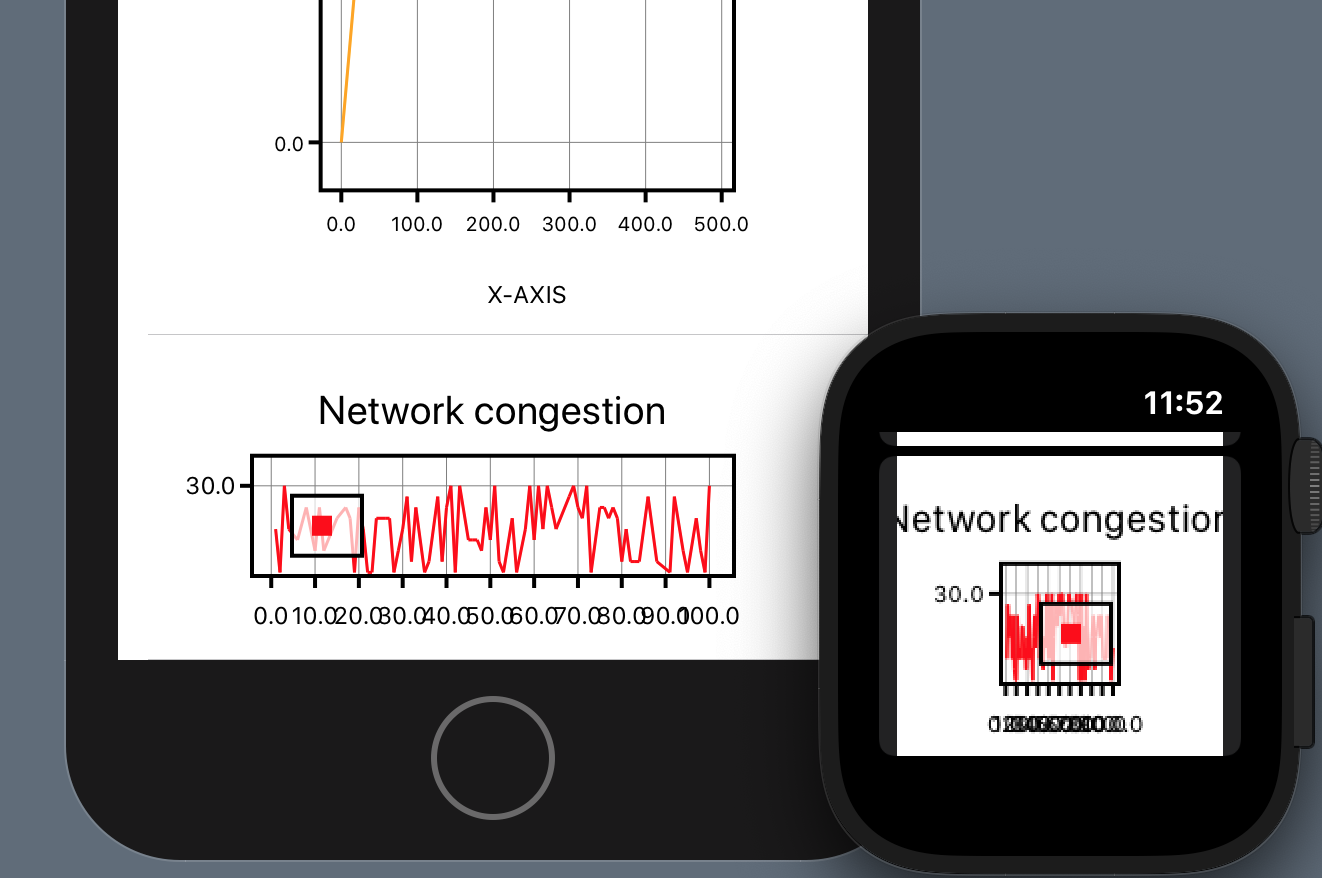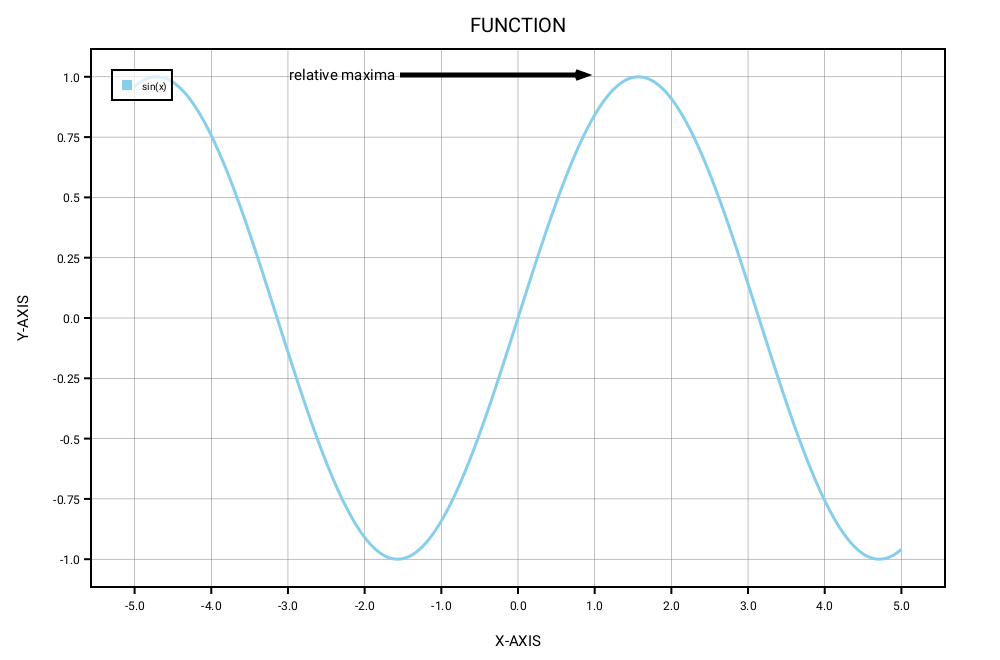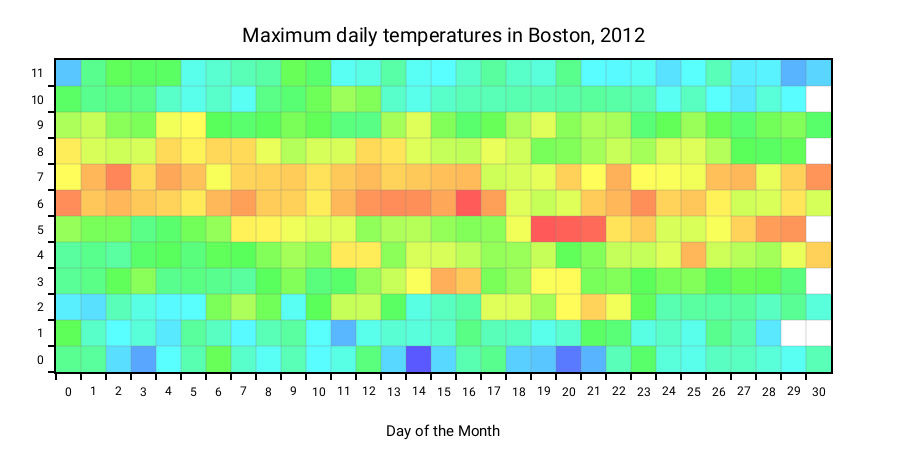## SwiftPlot

### 2.0.0

Swift library for Data Visualization :bar_chart:

### swiftplot 2.0.0

###### 2020-04-08T10:26:30Z

Welcome to the April 2020 release of swiftplot 2.0.0.

# Contributors

This release is the result of three months of work by the talented and dedicated swiftplot development team. Here is a list of the contributors in alphabetical order:

Interested in joining the swiftplot development team or want to get in touch with the developers? Take a look at our contribution guidelines. Just interested in following swiftplot's developement and future releases? Follow us on Twitter.

# Changelog

### ⭐️ iOS/tvOS/watchOS Support

swiftplot now has support for all apple platforms including macOS, iOS, tvOS and watchOS through the updated QuartzRenderer.### ⭐️ Annotations Support

swiftplot now supports the following plot annotations:

• Arrow
• Text
• Box
• Brackets### ⭐️ New Plot type: Heatmap

A new plot has been added `Heatmap<T>`, that can plot any sequence of any type.# Other bug fixes, changes and improvements

• Move examples to become tests.
• Simplify layout maths by adding `Rect` and `Size` primitives.
• Unify graph plot layout and implement new layout algorithm.
• Fix colors in QuartzRenderer.
• Add angled text drawing to QuartzRenderer.
• Use `butt` linecap style for consistency and fix solid lines that should be dashed in SVGRenderer.
• Add support for external CoreGraphics contexts (e.g. from AppKit/UIKit views).
• Clip LineCharts instead of clamping.
• Make SubPlot a Plot, therefore allowing the creation of nested SubPlots.
• Faster base64 encoding using a non-copying Data object.
• Verify that test output files actually match the reference files.
• Fix base64 PNG rendering.
• Rewrite the `drawData` implementation and implement better algorithm in Histogram to handle the previously unhandled edge cases.
• Explicitly set font smoothing to true in QuartzRenderer.
• Add Polygon and Polyline types.
• Use color alpha while drawing text in SVG Renderer.
• Fix draw line stroke thickness bug.
• Convert the plots to value types.
• Add new tutorial notebook: Machine Learning with Swift for TensorFlow and SwiftPlot
• LineGraph layout data calculation fixes.
• Add Support for Coordinate Conversion between different coordinate spaces.
• Update AGG version to 2.6.
• Add single renderer function to draw rectangles.
• Add Anchor Support for Annotations.
• Add instructions to setup Docker container for swift-jupyter and swiftplot.
• Add renderer function to draw solid ellipses.
• Performance improvements to recalculate bins in Histogram.

## Overview

The SwiftPlot framework is a cross-platform library that lets you plot graphs natively in Swift. The existing Swift plotting frameworks (such as CorePlot) run only on iOS or Mac. The idea behind SwiftPlot is to create a cross-platform library that runs on iOS, Mac, Linux and Windows.

SwiftPlot currently uses three rendering backends to generate plots:

• Anti-Grain Geometry(AGG) C++ rendering library
• A simple SVG Renderer
• A Core Graphics renderer with support for macOS, iOS, watchOS and tvOS

To encode the plots as PNG images it uses the lodepng library.
SwiftPlot can also be used in Jupyter Notebooks, with Python interop support for Google Colab.

Examples, demonstrating all the features, have been included with the repository under the `Tests/SwiftPlotTests` directory. To run the examples, clone the repository, and run the `swift test` command from the package directory.

Jupyter Notebook examples are under the `Notebooks` directory.

The resultant images are stored in a directory named `output`. The `Tests` folder includes a collection of reference images in the `Reference` directory.

SwiftPlot is licensed under `Apache 2.0`. View license

## How to include the library in your package

Add the library to your projects dependencies in the Package.swift file as shown below.

```dependencies: [
.package(url: "https://github.com/KarthikRIyer/swiftplot.git", from: "2.0.0")),
],```

In case you get an error saying that a file ft2build.h is not found, you need to install the freetype development package.

Linux

`sudo apt-get install libfreetype6-dev`

macOS

`brew install freetype`

If the above method doesn't work you can also build and install freetype on your own. You can find the source code and build instructions here.

## How to include the library in your Jupyter Notebook

Add these lines to the first cell:

```%install-swiftpm-flags -Xcc -isystem/usr/include/freetype2 -Xswiftc -lfreetype
%install '.package(url: "https://github.com/IBM-Swift/BlueCryptor.git", from: "1.0.28")' Cryptor
%install '.package(url: "https://github.com/KarthikRIyer/swiftplot", from: "2.0.0")' SwiftPlot AGGRenderer```

In order to display the generated plot in the notebook, add this line to a new cell:

`%include "EnableJupyterDisplay.swift"`

If you wish to display the generated plot in a Google Colab environment, add these lines to a new cell instead:

```import Python
%include "EnableIPythonDisplay.swift"
func display(base64EncodedPNG: String) {
let displayImage = Python.import("IPython.display")
let codecs = Python.import("codecs")
let imageData = codecs.decode(Python.bytes(base64EncodedPNG, encoding: "utf8"), encoding: "base64")
displayImage.Image(data: imageData, format: "png").display()
}```

Note that because Google Colab doesn't natively support Swift libraries that produce rich output, we use Swift's Python interop as a workaround.

## How to setup Docker instance for SwiftPlot

For computers running MacOS or Windows, Docker instance is to easy to setup and use `swift-jupyter`. Please refer SwiftPlot_Docker_setup.md for setup instructions.

## Examples

Here are some examples to provide you with a headstart to using this library. Here we will be looking at plots using only the AGGRenderer, but the procedure will remain the same for SVGRenderer. To use the library in your package, include it as a dependency to your target, in the Package.swift file.

More tests can be found in the SwiftPlotTests folder.

#### Simple Line Graph

```import SwiftPlot
import AGGRenderer

let x:[Float] = [10,100,263,489]
let y:[Float] = [10,120,500,800]

var agg_renderer: AGGRenderer = AGGRenderer()
var lineGraph = LineGraph<Float,Float>(enablePrimaryAxisGrid: true)
lineGraph.addSeries(x, y, label: "Plot 1", color: .lightBlue)
lineGraph.plotTitle.title = "SINGLE SERIES"
lineGraph.plotLabel.xLabel = "X-AXIS"
lineGraph.plotLabel.yLabel = "Y-AXIS"
lineGraph.plotLineThickness = 3.0
lineGraph.drawGraphAndOutput(fileName: filePath+"agg/"+fileName, renderer: agg_renderer)```#### Line Graph with multiple series of data

```import SwiftPlot
import AGGRenderer
import SVGRenderer

let x1:[Float] = [0,100,263,489]
let y1:[Float] = [0,320,310,170]
let x2:[Float] = [0,50,113,250]
let y2:[Float] = [0,20,100,170]

var agg_renderer: AGGRenderer = AGGRenderer()
var lineGraph = LineGraph<Float,Float>(enablePrimaryAxisGrid: true)
lineGraph.addSeries(x1, y1, label: "Plot 1", color: .lightBlue)
lineGraph.addSeries(x2, y2, label: "Plot 2", color: .orange)
lineGraph.plotTitle.title = "MULTIPLE SERIES"
lineGraph.plotLabel.xLabel = "X-AXIS"
lineGraph.plotlabel.yLabel = "Y-AXIS"
lineGraph.plotLineThickness = 3.0
lineGraph.drawGraphAndOutput(fileName: filePath+"agg/"+fileName, renderer: agg_renderer)```#### Line Graph with Sub Plots stacked horizontally

```import SwiftPlot
import AGGRenderer

let x:[Float] = [10,100,263,489]
let y:[Float] = [10,120,500,800]

var agg_renderer: AGGRenderer = AGGRenderer()
var subplot = SubPlot(layout: .horizontal)

var lineGraph1 = LineGraph<Float,Float>(enablePrimaryAxisGrid: true)
lineGraph1.addSeries(x, y, label: "Plot 1", color: .lightBlue)
lineGraph1.plotTitle.title = "PLOT 1"
lineGraph1.plotLabel.xLabel = "X-AXIS"
lineGraph1.plotLabel.yLabel = "Y-AXIS"
lineGraph1.plotLineThickness = 3.0

var lineGraph2 = LineGraph<Float,Float>(enablePrimaryAxisGrid: true)
lineGraph2.addSeries(x, y, label: "Plot 2", color: .orange)
lineGraph2.plotTitle.title = "PLOT 2"
lineGraph2.plotLabel.xLabel = "X-AXIS"
lineGraph2.plotLabel.yLabel = "Y-AXIS"
lineGraph2.plotLineThickness = 3.0

subplot.plots = [lineGraph1, lineGraph2]
subPlot.drawGraphAndOutput(fileName: "subPlotsHorizontallyStacked", renderer: agg_renderer)```#### Plot functions using LineGraph

```import Foundation
import SwiftPlot
import AGGRenderer

func function(_ x: Float)->Float {
return 1.0/x
}

var agg_renderer: AGGRenderer = AGGRenderer()
var lineGraph = LineGraph<Float,Float>(enablePrimaryAxisGrid: true)
lineGraph.addFunction(function, minX: -5.0, maxX: 5.0, numberOfSamples: 400, clampY: -50...50, label: "Function", color: .orange)
lineGraph.plotTitle.title = "FUNCTION"
lineGraph.plotLabel.xLabel = "X-AXIS"
lineGraph.plotLabel.yLabel = "Y-AXIS"
lineGraph.drawGraphAndOutput(fileName: "functionPlotLineGraph", renderer: agg_renderer)```#### Using a secondary axis in LineGraph

```import SwiftPlot
import AGGRenderer

let x:[Float] = [10,100,263,489]
let y:[Float] = [10,120,500,800]
let x1:[Float] = [100,200,361,672]
let y1:[Float] = [150,250,628,800]

var agg_renderer: AGGRenderer = AGGRenderer()
var lineGraph = LineGraph<Float,Float>()
lineGraph.addSeries(x1, y1, label: "Plot 1", color: .lightBlue, axisType: .primaryAxis)
lineGraph.addSeries(x, y, label: "Plot 2", color: .orange, axisType: .secondaryAxis)
lineGraph.plotTitle.title = "SECONDARY AXIS"
lineGraph.plotLabel.xLabel = "X-AXIS"
lineGraph.plotLabel.yLabel = "Y-AXIS"
lineGraph.plotLineThickness = 3.0
lineGraph.drawGraphAndOutput(fileName: filePath+"agg/"+fileName, renderer: agg_renderer)```

The series plotted on the secondary axis are drawn dashed.#### Displaying plots in Jupyter Notebook

You can display plots in Jupyter Notebook using only the AGGRenderer. To do so, create the plots as shown in the above examples and instead of using the `drawGraphAndOutput` function from LineGraph, use the `drawGraph` function, then get a base64 encoded image from the AGGRenderer and pass it to the display function as showm below:

```lineGraph.drawGraph(renderer: agg_renderer)
display(base64EncodedPNG: agg_renderer.base64Png())```

## How does this work

All the plotting code, utility functions, and necessary types are included in the SwiftPlot module. Each Renderer is implemented as a separate module. Each Renderer must have SwiftPlot as its dependency and must conform to the Renderer protocol defined in Renderer.swift in the SwiftPlot module. Each plot type is a generic that accepts data conforming to a protocol, FloatConvertible. At the moment FloatConvertible supports both Float and Double. The Renderer protocol defines all the necessary functions that a Renderer needs to implement. Each Plot must conform to the Plot protocol. At the moment this protocol defines the necessary variablse and functions that each Plot must implement in order to support SubPlots.

You can add series to the plots using their respective functions(`addSeries` for LineGraph). This is stored in as an array of Series objects. You can set other properties such as plotTitle, plotLabel, plotDimensions, etc. To actually generate the plot you need to call either the `drawGraph` or `drawGraphAndOutput` function. This calculates all the parameters necessary to generate the plots such as the coordinates of the border, scaled points to plot, etc. Then it sends over this information to the renderer which has functions to draw primitives like lines, rectangles and text.

In case the Renderer is in C++(here in the case of AGG), a C wrapper is written which is in turn wrapped in Swift.

In order to display the plots in Jupyter notebook, we encode the image(which is in the form of an RGB buffer) to a PNG image in memory and return the encoded image to the Swift code where it is stored as NSData. Then it is encoded to base64 and passed to the display function in swift-jupyter which finally displays the image.

## Documentation

### LineGraph<T: FloatConvertible, U: FloatConvertible>

Function Description
init(points: [Point], width: Float = 1000, height: Float = 660, enablePrimaryAxisGrid: Bool = false, enableSecondaryAxisGrid: Bool = false) Initialize a LineGraph with a set of points
init(width: Float = 1000, height: Float = 660, enablePrimaryAxisGrid: Bool = false,enableSecondaryAxisGrid: Bool = false) Initialize a LineGraph
addSeries(_ s: Series, axisType: Axis.Location = Axis.Location.primaryAxis) Add a series to the plot
addSeries(points p: [Point], label: String, color: Color = Color.lightBlue, axisType: Axis<T,U>.Location = Axis<T,U>.Location.primaryAxis) Add a series to the plot with a set of points, a label and a color for the series
addSeries(_ x: [Float], _ y: [Float], label: String, color: Color = Color.lightBlue, axisType: Axis<T,U>.Location = Axis<T,U>.Location.primaryAxis) Add a series to the plot with a set of x and y coordinates, a label and a color for the series
addSeries(_ y: [Float], label: String, color: Color = Color.lightBlue, axisType: Axis<T,U>.Location = Axis<T,U>.Location.primaryAxis) Add a series to the plot with only the y-coordinates. The x-coordinates are automatically enumerated [1, 2, 3, ...]
addFunction(_ function: (Float)->Float, minX: Float, maxX: Float, numberOfSamples: Int = 400, label: String, color: Color = Color.lightBlue, axisType: Axis.Location = Axis.Location.primaryAxis) Add a function to plot along with the range of x-coordinates over which to plot, number of samples of the function to take for plotting, a label, and color for the plot
drawGraphAndOutput(fileName name: String = "swift_plot_line_graph", renderer: Renderer) Generate the plot and save the resultant image
drawGraph(renderer: Renderer) Generate the plot in memory
drawGraphOutput(fileName name: String = "swift_plot_line_graph", renderer: Renderer) Save the generated plot to disk
Property
plotTitle: PlotTitle? = nil
plotLabel: PlotLabel? = nil
var plotDimensions: PlotDimensions
plotLineThickness: Float = 1.5
gridLineThickness: Float = 0.5
markerTextSize: Float = 12
gridColor: Color = .gray

### BarChart<T: LosslessStringConvertible, U: FloatConvertible>

Function Description
init(width: Float = 1000, height: Float = 660, enableGrid: Bool = false) Initialize a BarChart
addStackSeries(_ x: [U], label: String, color: Color = .lightBlue, hatchPattern: BarGraphSeriesOptions.Hatching = .none) Add a stacked series to the plot
addSeries(values: [Pair<T,U>], label: String, color: Color = Color.lightBlue, hatchPattern: BarGraphSeriesOptions.Hatching = .none, graphOrientation: BarGraph.GraphOrientation = .vertical) Add a series to the plot using a Pair array
addSeries(_ x: [T], _ y: [U], label: String, color: Color = Color.lightBlue, hatchPattern: BarGraphSeriesOptions.Hatching = .none, graphOrientation: BarGraph.GraphOrientation = .vertical) Add a series to the plot using a Pair array
drawGraphAndOutput(fileName name: String = "swift_plot_bar_graph", renderer: Renderer) Generate the plot and save the resultant image
drawGraph(renderer: Renderer) Generate the plot in memory
drawGraphOutput(fileName name: String = "swift_plot_bar_graph", renderer: Renderer) Save the generated plot to disk
Property
plotTitle: PlotTitle? = nil
plotLabel: PlotLabel? = nil
var plotDimensions: PlotDimensions
space: Int = 20 (Sets the space between two bars)
gridLineThickness: Float = 0.5
markerTextSize: Float = 12
gridColor: Color = .gray

### Histogram<T:FloatConvertible>

Function Description
init(width: Float = 1000, height: Float = 660, isNormalized: Bool = false, enableGrid: Bool = false) Initialize a Histogram
addSeries(data: [T], bins: Int, label: String, color: Color = .lightBlue, histogramType: HistogramSeriesOptions.HistogramType = .bar) Add a series using an array.
addStackSeries(data: [T], label: String, color: Color = .lightBlue) Add a stacked series to the plot
drawGraphAndOutput(fileName name: String = "swift_plot_histogram", renderer: Renderer) Generate the plot and save the resultant image
drawGraph(renderer: Renderer) Generate the plot in memory
drawGraphOutput(fileName name: String = "swift_plot_histogram", renderer: Renderer) Save the generated plot to disk
Property
plotTitle: PlotTitle? = nil
plotLabel: PlotLabel? = nil
var plotDimensions: PlotDimensions
var strokeWidth: Float = 2
gridLineThickness: Float = 0.5
markerTextSize: Float = 12
gridColor: Color = .gray

### ScatterPlot<T:FloatConvertible, U:FloatConvertible>

Function Description
init(width: Float = 1000, height: Float = 660, isNormalized: Bool = false, enableGrid: Bool = false) Initialize a ScatterPlot.
init(points p: [Pair<T,U>], width: Float = 1000, height: Float = 660, enableGrid: Bool = false) Initialize a ScatterPlot.
addSeries(points: [Pair<T,U>], label: String, color: Color = .lightBlue, scatterPattern: ScatterPlotSeriesOptions.ScatterPattern = .circle) Add a series using an array of Pairs.
addSeries(_ x: [T], _ y: [U], label: String, color: Color = .lightBlue, scatterPattern: ScatterPlotSeriesOptions.ScatterPattern = .circle) Add a series using separate x and y arrays.
addSeries(_ x: [T], _ y: [U], label: String, startColor: Color = .lightBlue, endColor: Color = .lightBlue, scatterPattern: ScatterPlotSeriesOptions.ScatterPattern = .circle)) Add a series using separate x and y arrays and specify a start and end color for the scatter points.
addSeries(_ y: [U], label: String, color: Color = .lightBlue, scatterPattern: ScatterPlotSeriesOptions.ScatterPattern = .circle) Add a series using just the y array. It will be plotted against the index of the point.
addSeries(_ y: [U], label: String, startColor: Color = .lightBlue, endColor: Color = .lightBlue, scatterPattern: ScatterPlotSeriesOptions.ScatterPattern = .circle) Add a series using just the y array. It will be plotted against the index of the point. Also specify the start and end color for the scatter points.
drawGraphAndOutput(fileName name: String = "swift_plot_scatter_plot", renderer: Renderer) Generate the plot and save the resultant image
drawGraph(renderer: Renderer) Generate the plot in memory
drawGraphOutput(fileName name: String = "swift_plot_scatter_plot", renderer: Renderer) Save the generated plot to disk
Property
plotTitle: PlotTitle? = nil
plotLabel: PlotLabel? = nil
plotDimensions: PlotDimensions
scatterPatternSize: Float = 10
gridLineThickness: Float = 0.5
markerTextSize: Float = 12
gridColor: Color = .gray

### SubPlot

enum stackPattern (to be passed in place of stackPattern in the initializer)
verticallyStacked
horizontallyStacked
gridStacked
Function Description
init(width: Float = 1000, height: Float = 660, numberOfPlots n: Int = 1, numberOfRows nR: Int = 1, numberOfColumns nC: Int = 1, stackPattern: Int = 0) Initialize a SubPlot
draw(plots: [Plot], renderer: Renderer, fileName: String = "subPlot_output") Generate plot with the plots passed in as Sub Plots and save the image to disk

### PlotDimensions

Function Description
init(frameWidth : Float = 1000, frameHeight : Float = 660) Create a PlotDimensions variable with a frame width and height

### Pair<T,U>

Property
x: T
y: U
Function Description
init(_ x: T, _ y: T) Create a Pair using x and y
typealias
Point = Pair<Float, Float>
Property
zeroPoint = Point(0.0, 0.0)

### PlotLabel

Property
xLabel: String = "X-Axis"
yLabel: String = "Y-Axis"
labelSize: Float = 15
xLabelLocation = zeroPoint
yLabelLocation = zeroPoint

### PlotTitle

Property
title : String = "TITLE"
titleSize : Float = 15
titleLocation = zeroPoint

### Color

Function Description
init(_ r: Float, _ g: Float, _ b: Float, _ a: Float) Create a Color with r, g, b and a values. Each of them being between 0.0 and 1.0
Property(only on macOS and iOS)
cgColor: CGColor (return the corresponding CGColor)

Built-in Colors can be found here.

### PlotLabel

Property
xLabel: String = "X-Axis"
yLabel: String = "Y-Axis"
labelSize: Float = 15
xLabelLocation = zeroPoint
yLabelLocation = zeroPoint

### Axis<T,U>

enum Location (to be passed into addSeries function in LineGraph)
primaryAxis
secondaryAxis

### ScatterPlotSeriesOptions

enum ScatterPattern (to be passed into addSeries function in ScatterPlot)
circle
square
triangle
diamond
hexagon
pentagon
star

### HistogramSeriesOptions

enum HistogramType: CaseIterable (to be passed into addSeries function in Histogram)
bar
step

### BarGraphSeriesOptions

enum Hatching: Int, CaseIterable (to be passed into addSeries function in BarGraph)
none = 0
forwardSlash = 1
backwardSlash = 2
hollowCircle = 3
filledCircle = 4
vertical = 5
horizontal = 6
grid = 7
cross = 8

### Base64Encoder

Function Description
encodeBase64PNG(pngBufferPointer: UnsafePointer, bufferSize: Int) -> String Encode a PNG image into base64 format.

## Limitations

• FloatConvertible supports only Float and Double. We plan to extend this to Int in the future.

## Guidelines for Contributors

If you want to contribute to improve this library, please read our guidelines. Feel free to open an issue.

## Credits

1. Maxim Shemanarev : The AGG library is directly used to render plots.
2. Lode Vandevenne : The lodepng library is directly used to encode PNG images.
3. The FreeType Project : AGG uses FreeType to draw text.
4. Brad Larson and Marc Rasi for their invaluable guidance.

### Description

• Swift Tools 5.1.0

### Dependencies

• None
Last updated: Tue Jan 17 2023 00:57:01 GMT-0500 (GMT-05:00)